{{announcement.body}}
{{announcement.title}}

# Example of Deep Learning With R and Keras

DZone 's Guide to

# Example of Deep Learning With R and Keras

### Recreate the solution that one dev created for the Carvana Image Masking Challenge, which involved using AI and image recognition to separate photographs of cars.

· AI Zone ·
Free Resource

Comment (0)

Save
{{ articles.views | formatCount}} Views

Users of R have long been deprived of the opportunity to join the deep learning movement while remaining within the same programming language. With the release of MXNet, the situation began to change, but the frequent updates to the original documentation and changes that break backward compatibility still limit the popularity of this library.

The use of R interfaces for TensorFlow and Keras with backends for choice (i.e. TensorFlow, Theano, CNTK) combined with detailed documentation and a lot of examples looks much more attractive. This article presents a solution to the problem of segmenting images in Carvana Image Masking Challenge, in which you want to learn how to separate cars photographed from 16 different angles will be dismantled. (You can learn about the winners here.) The neural network part is fully implemented on Keras, image processing is answered by magick (interface to ImageMagick), and parallel processing is provided by parallel + `doParallel` +  `foreach` (Windows) or parallel + `doMC` +  `foreach` (Linux).

## What Do We Need to Install?

We assume that the reader already has a GPU from Nvidia with ≥4 GB of memory (it can be less, but it will not be so interesting), and also the CUDA and cuDNN libraries are installed. For Linux, installing the latter is easy, and for Windows, even easier! (See the CUDA & cuDNN section of the manual.)

Further, it is desirable to install the Anaconda distribution with Python 3. To save space, you can use Miniconda. If suddenly the version of Python in the distribution is ahead of the last version supported by Tensorflow, you can replace it with a command of the form `conda install python = 3.6`. Also, everything will work with the usual Python and virtual environments.

The list of used R packages is as follows:

List of packages for Windows:

``````library(keras)
library(magick)
library(abind)
library(reticulate)
library(parallel)
library(doParallel)
library(foreach)``````

List of packages for Linux:

``````library(keras)
library(magick)
library(abind)
library(reticulate)
library(parallel)
library(doMC)
library(foreach)``````

All of them are installed with CRAN, but it's better to take Keras with GitHub: (`devtools:: install_github ("rstudio/keras"`). The subsequent run of the `install_keras ()` command will create a conda environment and install the correct versions of Python's Tensorflow and Keras. If this command for some reason refuses to work correctly (for example, could not find the required Python distribution) or specific versions of the used libraries are required, you should create a conda environment yourself, install the necessary packages in it, and then in R, specify that the environment is using the `use_condaenv ()` command.

Here's a list of the parameters used:

``````input_size <- 128 # Width and height of images supplied to the input of a neural network

epochs <- 30 # The number of epochs
batch_size <- 16 # Batch size

orig_width <- 1918 # Original image width
orig_height <- 1280 # Height of source images

train_samples <- 5088 # Size of training sample
train_index <- sample (1: train_samples, round (train_samples * 0.8)) # 80%
val_index <- c (1: train_samples) [- train_index]

# Folders with pictures
images_dir <- "input / train /"

## Working With Images: magick as an Alternative to OpenCV

When solving the task of machine learning on graphics data, one must be able, at the very least, to read images from a disk and transfer them to a neural network in the form of arrays. Typically, you also need to be able to perform a variety of image transformations in order to implement augmentation — the addition of a training sample by artificial examples created from samples present in the training sample itself. Augmentation is (almost) always able to increase the quality of the model. Looking ahead, we should note that all this needs to be done quickly and in a multithreaded fashion — even on a relatively fast CPU and a relatively slow video card, the preparatory stage may turn out to be more resource-intensive than the actual learning of the neural network.

In Python, OpenCV is traditionally used to work with images. Versions of this mega library for R have not yet been created, and calling its functions through reticulate looks like an unsportsmanlike solution, so we will choose from available alternatives. The top three most powerful graphics packages are as follows:

1. EBImage: The package is created using S4-classes and placed in the repository Bioconductor, which implies the highest quality requirements for both the package itself and its documentation. Unfortunately, enjoying the vast possibilities of this software product is hampered by its extremely low speed.

2. imager: This package looks more interesting in terms of performance since the main work in it is performed by the compiled code in the face of the CImg library. Among the merits, we can note the support of the "pipeline" operator (and other operators from magrittr) and close integration with packages from it. It uses tidyverse, including ggplot2, as well as support for the split-apply-combine ideology. Only an incomprehensible bug makes the functions for reading pictures on some PCs inoperable, preventing the author of this message from stopping their choice of this package.

3. magick is a wrapper package for ImageMagick, actively developed by rOpenSci community members. It combines all the advantages of the previous package, including stability and killer features (useless in the framework of our task) in the form of integration with the OCR-library Tesseract. Measurements of speed, when reading and transforming pictures on a different number of cores, are given below. Of the minuses, esoteric syntax can be noted; for example, for cutting or resizing, you need to pass a string of the form "100x150 + 50" instead of the usual arguments of type height and width. And since our auxiliary functions for preprocessing will be parametrized just by these values, you will have to use the ugly `paste0 (...)` or `sprint (...)` designs

We will reproduce the solution of the Kaggle Carvana Image Masking Challenge from Peter-a Giannakopoulos.

You need to read the files in pairs — an image and the corresponding mask — and apply the same transformations (rotations, shifts, reflections, changes of scale) using the augmentation to the image and the mask. We implement reading in the form of one function, which will immediately reduce the images to the desired size:

``````imagesRead <- function(image_file,
target_width = 128,
target_height = 128) {
img <- image_scale(img, paste0(target_width, "x", target_height, "!"))

}``````

The result of the function with the mask applied to the image:

``````img <- "input/train/0cdf5b5d0ce1_01.jpg"

target_width = 400,
target_height = 400)

image_composite(x_y_imgs\$img,
operator = "blend",
compose_args = "60") %>%
image_write(path = "pics/pic1.jpg", format = "jpg")``````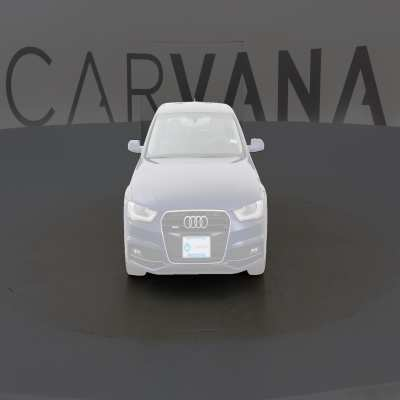The first kind of augmentation will be changing the brightness (saturation), saturation (duration), and tone (hue). For obvious reasons, it applies to a colored image, but not to a black and white mask:

``````randomBSH <- function(img,
u = 0,
brightness_shift_lim = c(90, 110), # percentage
saturation_shift_lim = c(95, 105), # of current value
hue_shift_lim = c(80, 120)) {

if (rnorm(1) < u) return(img)

brightness_shift <- runif(1,
brightness_shift_lim,
brightness_shift_lim)
saturation_shift <- runif(1,
saturation_shift_lim,
saturation_shift_lim)
hue_shift <- runif(1,
hue_shift_lim,
hue_shift_lim)

img <- image_modulate(img,
brightness = brightness_shift,
saturation =  saturation_shift,
hue = hue_shift)
img
}``````

This conversion is applied with a probability of 50% (half the original image will be returned if `(rnorm (1) <u) return (img)`); the value of the change of each of the three parameters is selected randomly within the range of values specified in percentage of the original values.

Also, with a 50% probability, we will use horizontal reflections of the image and mask:

``````randomHorizontalFlip <- function(img,
u = 0) {

}``````

Result:

``````img <- "input/train/0cdf5b5d0ce1_01.jpg"

target_width = 400,
target_height = 400)
x_y_imgs\$img <- randomBSH(x_y_imgs\$img)
x_y_imgs <- randomHorizontalFlip(x_y_imgs\$img,
image_composite(x_y_imgs\$img,
operator = "blend",
compose_args = "60") %>%
image_write(path = "pics/pic2.jpg", format = "jpg")``````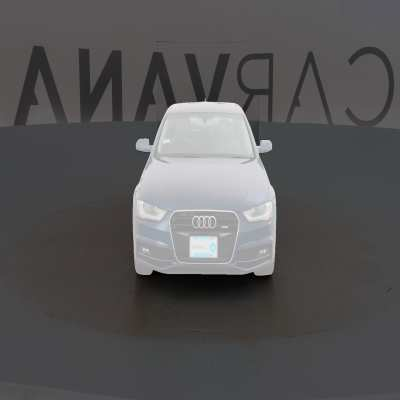The rest of the transformations, for the sake of further exposition, are not fundamental; therefore, we shall not dwell on them. The last stage is the transformation of pictures into arrays:

``````img2arr <- function(image,
target_width = 128,
target_height = 128) {
result <- aperm(as.numeric(image[])[, , 1:3], c(2, 1, 3)) # transpose
dim(result) <- c(1, target_width, target_height, 3)
return(result)
}

target_width = 128,
target_height = 128) {
result <- t(as.numeric(mask[])[, , 1]) # transpose
dim(result) <- c(1, target_width, target_height, 1)
return(result)
}``````

Transposition is necessary in order for the image lines to retain rows in the matrix; the image is formed line-by-line (as the scanning beam moves in the tube), while the matrices in R are filled in columns (column-major, or Fortran-style; for comparison, in numpy, you can switch between column-major and row-major formats). You can do without it, but it's clearer to have it.

## Parallel execution of the R Code in Windows and Linux

A general idea of parallel computations in R can be found in the Package 'Parallel' manuals, Getting Started With doParallel and foreach, and Getting Started with doMC and foreach. The algorithm is as follows. Start the cluster with the required number of cores:

``cl <- makePSOCKcluster(4) # doParallel``

SOCK-clusters are a universal solution, allowing, among other things, using the CPU of several PCs. Unfortunately, our example with iterators and neural network training works under Windows but refuses to work under Linux. In Linux, you can use the alternative `doMC` package, which creates clusters using the forks of the source process. The remaining steps do not need to be done.

Both `doParallel` and `doMC` serve as intermediaries between the parallel and `foreach` functionality.

When using  `makePSOCKcluster ()`, you need to load the necessary packages and functions inside the cluster:

``````clusterEvalQ(cl, {
library(magick)
library(abind)
library(reticulate)

target_width = 128,
target_height = 128) {
img <- image_scale(img, paste0(target_width, "x", target_height, "!"))

}

randomBSH <- function(img,
u = 0,
brightness_shift_lim = c(90, 110), # percentage
saturation_shift_lim = c(95, 105), # of current value
hue_shift_lim = c(80, 120)) {

if (rnorm(1) < u) return(img)

brightness_shift <- runif(1,
brightness_shift_lim,
brightness_shift_lim)
saturation_shift <- runif(1,
saturation_shift_lim,
saturation_shift_lim)
hue_shift <- runif(1,
hue_shift_lim,
hue_shift_lim)

img <- image_modulate(img,
brightness = brightness_shift,
saturation =  saturation_shift,
hue = hue_shift)
img
}

randomHorizontalFlip <- function(img,
u = 0) {

}

img2arr <- function(image,
target_width = 128,
target_height = 128) {
result <- aperm(as.numeric(image[])[, , 1:3], c(2, 1, 3)) # transpose
dim(result) <- c(1, target_width, target_height, 3)
return(result)
}

target_width = 128,
target_height = 128) {
result <- t(as.numeric(mask[])[, , 1]) # transpose
dim(result) <- c(1, target_width, target_height, 1)
return(result)
}
})``````

We register the cluster as a parallel backend for foreach:

``registerDoParallel(cl)``

After that, you can run the code in parallel mode:

``````imgs <- list.files("input/train/",
pattern = ".jpg",
full.names = TRUE)[1:16]
pattern = ".png",
full.names = TRUE)[1:16]

x_y_batch <- foreach(i = 1:16) %dopar% {
# augmentation
x_y_imgs\$img <- randomBSH(x_y_imgs\$img)
x_y_imgs <- randomHorizontalFlip(x_y_imgs\$img,
# return as arrays
x_y_arr <- list(x = img2arr(x_y_imgs\$img),
}
str(x_y_batch)
# List of 16
#  \$ :List of 2
#   ..\$ x: num [1, 1:128, 1:128, 1:3] 0.953 0.957 0.953 0.949 0.949 ...
#   ..\$ y: num [1, 1:128, 1:128, 1] 0 0 0 0 0 0 0 0 0 0 ...
#  \$ :List of 2
#   ..\$ x: num [1, 1:128, 1:128, 1:3] 0.949 0.957 0.953 0.949 0.949 ...
#   ..\$ y: num [1, 1:128, 1:128, 1] 0 0 0 0 0 0 0 0 0 0 ...
# ....``````

In the end, do not forget to stop the cluster:

``stopCluster(cl)``

With the help of the microbenchmark package, we will check the benefits of using several cores/threads. On a GPU with 4 GB of memory, you can work with batches of 16 pairs of images, so it's advisable to use two, four, eight, or 16 streams (the time is in seconds):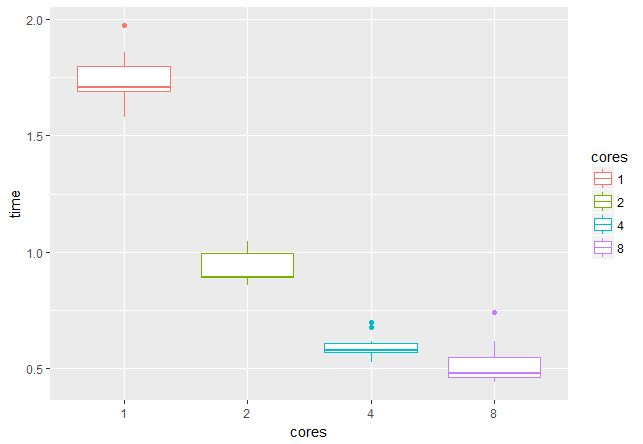On 16 streams, it was not possible to check, but it is clear that when moving from one to four streams, the speed increases about 3x — which is very encouraging.

## Reticulate and Iterator

To work with data that does not fit in memory, we use iterators from the `reticulate` package. The basis is the usual function-closure; that is, a function that, when called, returns another function together with the calling environment.

`train_generator`:

``````train_generator <- function(images_dir,
samples_index,
batch_size) {

images_iter <- list.files(images_dir,
pattern = ".jpg",
full.names = TRUE)[samples_index] # for current epoch
images_all <- list.files(images_dir,
pattern = ".jpg",
full.names = TRUE)[samples_index]  # for next epoch
pattern = ".gif",
full.names = TRUE)[samples_index] # for current epoch
pattern = ".gif",
full.names = TRUE)[samples_index] # for next epoch

function() {

# start new epoch
if (length(images_iter) < batch_size) {
images_iter <<- images_all
}

batch_ind <- sample(1:length(images_iter), batch_size)

batch_images_list <- images_iter[batch_ind]
images_iter <<- images_iter[-batch_ind]

x_y_batch <- foreach(i = 1:batch_size) %dopar% {
# augmentation
x_y_imgs\$img <- randomBSH(x_y_imgs\$img)
x_y_imgs <- randomHorizontalFlip(x_y_imgs\$img,
# return as arrays
x_y_arr <- list(x = img2arr(x_y_imgs\$img),
}

x_y_batch <- purrr::transpose(x_y_batch)

x_batch <- do.call(abind, c(x_y_batch\$x, list(along = 1)))

y_batch <- do.call(abind, c(x_y_batch\$y, list(along = 1)))

result <- list(keras_array(x_batch),
keras_array(y_batch))
return(result)
}
}``````

`val_generator`:

``````val_generator <- function(images_dir,
samples_index,
batch_size) {
images_iter <- list.files(images_dir,
pattern = ".jpg",
full.names = TRUE)[samples_index] # for current epoch
images_all <- list.files(images_dir,
pattern = ".jpg",
full.names = TRUE)[samples_index]  # for next epoch
pattern = ".gif",
full.names = TRUE)[samples_index] # for current epoch
pattern = "gif",
full.names = TRUE)[samples_index] # for next epoch

function() {

# start new epoch
if (length(images_iter) < batch_size) {
images_iter <<- images_all
}

batch_ind <- sample(1:length(images_iter), batch_size)

batch_images_list <- images_iter[batch_ind]
images_iter <<- images_iter[-batch_ind]

x_y_batch <- foreach(i = 1:batch_size) %dopar% {
# without augmentation

# return as arrays
x_y_arr <- list(x = img2arr(x_y_imgs\$img),
}

x_y_batch <- purrr::transpose(x_y_batch)

x_batch <- do.call(abind, c(x_y_batch\$x, list(along = 1)))

y_batch <- do.call(abind, c(x_y_batch\$y, list(along = 1)))

result <- list(keras_array(x_batch),
keras_array(y_batch))
return(result)
}
}``````

Here, in the environment of the call, the lists of processed files decreasing during each epoch are stored, as well as copies of the full lists that are used at the beginning of each subsequent epoch. In this implementation, you do not have to worry about accidentally mixing files — each batch is obtained by random sampling.

As shown above, `x_y_batch` is a list of 16 lists, each of which is a list of two arrays. The `purrr:: transpose ()` function turns the nested lists inside out, and we get a list of two lists, each of which is a list of 16 arrays. `and ()` combines arrays along the specified dimension, while `do.call ()` passes an arbitrary number of arguments to the internal function. Additional arguments ( `along = 1`) are set in a very bizarre way: ` do.call (and, c (x_y_batch \$ x, list (along = 1)))`.

It remains to turn these functions into objects understandable for Keras using `py_iterator ()`:

``````train_iterator <- py_iterator(train_generator(images_dir = images_dir,
samples_index = train_index,
batch_size = batch_size))

val_iterator <- py_iterator(val_generator(images_dir = images_dir,
samples_index = val_index,
batch_size = batch_size))``````

Calling `iter_next (train_iterator)` will return the result of one iteration, which is useful in the debugging phase.

## Segmentation and the Loss Function

The task of segmentation can be considered as a per-pixel classification: it predicts whether each pixel belongs to a particular class. For the case of two classes, the result will be a mask; if there are more than two classes, the number of masks will be equal to the number of classes minus 1 (analog of one-hot encoding). In our class competition, for only two (the machine and the background), the metric of quality is the dice-coefficient. He calculates this:

``````K <- backend()

dice_coef <- function(y_true, y_pred, smooth = 1.0) {
y_true_f <- K\$flatten(y_true)
y_pred_f <- K\$flatten(y_pred)
intersection <- K\$sum(y_true_f * y_pred_f)
result <- (2 * intersection + smooth) /
(K\$sum(y_true_f) + K\$sum(y_pred_f) + smooth)
return(result)
}``````

We will optimize the loss function, which is the sum of cross entropy and 1 - `dice_coef`:

``````bce_dice_loss <- function(y_true, y_pred) {
result <- loss_binary_crossentropy(y_true, y_pred) +
(1 - dice_coef(y_true, y_pred))
return(result)
}``````

## U-Net Architecture

U-Net is a classic architecture for solving segmentation problems. Here's a schematic diagram: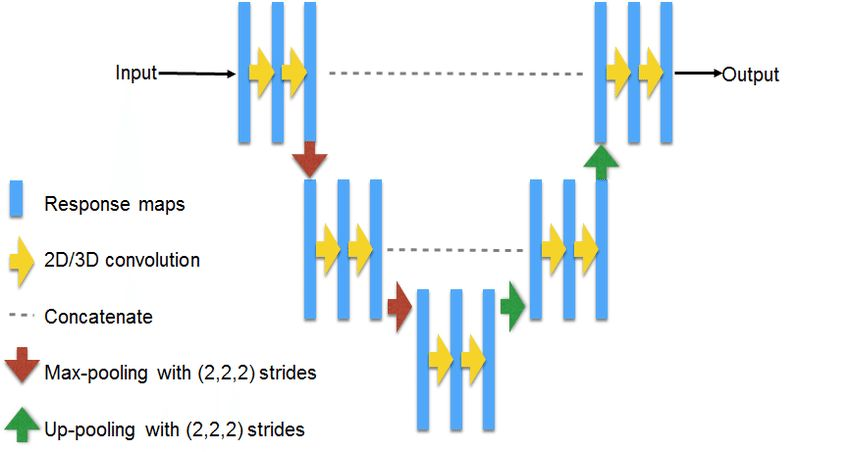Source

Following is the implementation for pictures 128x128.

U-Net 128:

``````get_unet_128 <- function(input_shape = c(128, 128, 3),
num_classes = 1) {

inputs <- layer_input(shape = input_shape)
# 128

down1 <- inputs %>%
layer_conv_2d(filters = 64, kernel_size = c(3, 3), padding = "same") %>%
layer_batch_normalization() %>%
layer_activation("relu") %>%
layer_conv_2d(filters = 64, kernel_size = c(3, 3), padding = "same") %>%
layer_batch_normalization() %>%
layer_activation("relu")
down1_pool <- down1 %>%
layer_max_pooling_2d(pool_size = c(2, 2), strides = c(2, 2))
# 64

down2 <- down1_pool %>%
layer_conv_2d(filters = 128, kernel_size = c(3, 3), padding = "same") %>%
layer_batch_normalization() %>%
layer_activation("relu") %>%
layer_conv_2d(filters = 128, kernel_size = c(3, 3), padding = "same") %>%
layer_batch_normalization() %>%
layer_activation("relu")
down2_pool <- down2 %>%
layer_max_pooling_2d(pool_size = c(2, 2), strides = c(2, 2))
# 32

down3 <- down2_pool %>%
layer_conv_2d(filters = 256, kernel_size = c(3, 3), padding = "same") %>%
layer_batch_normalization() %>%
layer_activation("relu") %>%
layer_conv_2d(filters = 256, kernel_size = c(3, 3), padding = "same") %>%
layer_batch_normalization() %>%
layer_activation("relu")
down3_pool <- down3 %>%
layer_max_pooling_2d(pool_size = c(2, 2), strides = c(2, 2))
# 16

down4 <- down3_pool %>%
layer_conv_2d(filters = 512, kernel_size = c(3, 3), padding = "same") %>%
layer_batch_normalization() %>%
layer_activation("relu") %>%
layer_conv_2d(filters = 512, kernel_size = c(3, 3), padding = "same") %>%
layer_batch_normalization() %>%
layer_activation("relu")
down4_pool <- down4 %>%
layer_max_pooling_2d(pool_size = c(2, 2), strides = c(2, 2))
# 8

center <- down4_pool %>%
layer_conv_2d(filters = 1024, kernel_size = c(3, 3), padding = "same") %>%
layer_batch_normalization() %>%
layer_activation("relu") %>%
layer_conv_2d(filters = 1024, kernel_size = c(3, 3), padding = "same") %>%
layer_batch_normalization() %>%
layer_activation("relu")
# center

up4 <- center %>%
layer_upsampling_2d(size = c(2, 2)) %>%
{layer_concatenate(inputs = list(down4, .), axis = 3)} %>%
layer_conv_2d(filters = 512, kernel_size = c(3, 3), padding = "same") %>%
layer_batch_normalization() %>%
layer_activation("relu") %>%
layer_conv_2d(filters = 512, kernel_size = c(3, 3), padding = "same") %>%
layer_batch_normalization() %>%
layer_activation("relu") %>%
layer_conv_2d(filters = 512, kernel_size = c(3, 3), padding = "same") %>%
layer_batch_normalization() %>%
layer_activation("relu")
# 16

up3 <- up4 %>%
layer_upsampling_2d(size = c(2, 2)) %>%
{layer_concatenate(inputs = list(down3, .), axis = 3)} %>%
layer_conv_2d(filters = 256, kernel_size = c(3, 3), padding = "same") %>%
layer_batch_normalization() %>%
layer_activation("relu") %>%
layer_conv_2d(filters = 256, kernel_size = c(3, 3), padding = "same") %>%
layer_batch_normalization() %>%
layer_activation("relu") %>%
layer_conv_2d(filters = 256, kernel_size = c(3, 3), padding = "same") %>%
layer_batch_normalization() %>%
layer_activation("relu")
# 32

up2 <- up3 %>%
layer_upsampling_2d(size = c(2, 2)) %>%
{layer_concatenate(inputs = list(down2, .), axis = 3)} %>%
layer_conv_2d(filters = 128, kernel_size = c(3, 3), padding = "same") %>%
layer_batch_normalization() %>%
layer_activation("relu") %>%
layer_conv_2d(filters = 128, kernel_size = c(3, 3), padding = "same") %>%
layer_batch_normalization() %>%
layer_activation("relu") %>%
layer_conv_2d(filters = 128, kernel_size = c(3, 3), padding = "same") %>%
layer_batch_normalization() %>%
layer_activation("relu")
# 64

up1 <- up2 %>%
layer_upsampling_2d(size = c(2, 2)) %>%
{layer_concatenate(inputs = list(down1, .), axis = 3)} %>%
layer_conv_2d(filters = 64, kernel_size = c(3, 3), padding = "same") %>%
layer_batch_normalization() %>%
layer_activation("relu") %>%
layer_conv_2d(filters = 64, kernel_size = c(3, 3), padding = "same") %>%
layer_batch_normalization() %>%
layer_activation("relu") %>%
layer_conv_2d(filters = 64, kernel_size = c(3, 3), padding = "same") %>%
layer_batch_normalization() %>%
layer_activation("relu")
# 128

classify <- layer_conv_2d(up1,
filters = num_classes,
kernel_size = c(1, 1),
activation = "sigmoid")

model <- keras_model(
inputs = inputs,
outputs = classify
)

model %>% compile(
optimizer = optimizer_rmsprop(lr = 0.0001),
loss = bce_dice_loss,
metrics = c(dice_coef)
)

return(model)
}

model <- get_unet_128()``````

The curly brackets in `{layer_concatenate (inputs = list (down4,.), Axis = 3)}` are needed to substitute the object in the form of the required argument, and not as the first one, as `otherwise%>%` does. You can suggest many modifications of this architecture; use `layer_conv_2d_transpose` instead of `layer_upsampling_2d`, apply separate convolutions in `layer_separable_conv_2d` instead of usual ones, and experiment with filter numbers and optimizer settings. There are options for resolutions up to 1024x1024, which are also easily ported to R.

In our model, there are many parameters:

``````# Total params: 34,540,737
# Trainable params: 34,527,041
# Non-trainable params: 13,696``````

## Training Models

Everything is simple. Run `tensorboard`:

``tensorboard("logs_r")``

As an alternative, the `tfruns` package is available, which adds an analog Tensorboard to the RStudio IDE and allows you to streamline the work on learning neural networks.

Specify the callback-and. We will use the early stop, reduce the speed of training when entering the plateau, and save the weight of the best model:

``````callbacks_list <- list(
callback_tensorboard("logs_r"),
callback_early_stopping(monitor = "val_python_function",
min_delta = 1e-4,
patience = 8,
verbose = 1,
mode = "max"),
callback_reduce_lr_on_plateau(monitor = "val_python_function",
factor = 0.1,
patience = 4,
verbose = 1,
epsilon = 1e-4,
mode = "max"),
callback_model_checkpoint(filepath = "weights_r/unet128_{epoch:02d}.h5",
monitor = "val_python_function",
save_best_only = TRUE,
save_weights_only = TRUE,
mode = "max" )
)``````

We start training and wait. On the GTX 1050ti, one era takes about ten minutes:

``````model %>% fit_generator(
train_iterator,
steps_per_epoch = as.integer(length(train_index) / batch_size),
epochs = epochs,
validation_data = val_iterator,
validation_steps = as.integer(length(val_index) / batch_size),
verbose = 1,
callbacks = callbacks_list
)``````

## Predictions Based on the Model

The spoiler provides a demo version of the prediction code using run-length encoding:

``````test_dir <- "input/test/"
test_samples <- 100064
test_index <- sample(1:test_samples, 1000)

target_width = 128,
target_height = 128) {
img <- image_scale(img, paste0(target_width, "x", target_height, "!"))
}

img2arr <- function(image,
target_width = 128,
target_height = 128) {
result <- aperm(as.numeric(image[])[, , 1:3], c(2, 1, 3)) # transpose
dim(result) <- c(1, target_width, target_height, 3)
return(result)
}

arr2img <- function(arr,
target_width = 1918,
target_height = 1280) {
img <- image_scale(img, paste0(target_width, "x", target_height, "!"))
}

dim(img) <- c(128, 128, 1)
img <- arr2img(img)
arr <- as.numeric(img[])[, , 2]
vect <- ifelse(as.vector(arr) >= 0.5, 1, 0)
turnpoints <- c(vect, 0) - c(0, vect)
starts <- which(turnpoints == 1)
ends <- which(turnpoints == -1)
paste(c(rbind(starts, ends - starts)), collapse = " ")
}

cl <- makePSOCKcluster(4)
clusterEvalQ(cl, {
library(magick)
library(abind)
library(reticulate)

target_width = 128,
target_height = 128) {
img <- image_scale(img, paste0(target_width, "x", target_height, "!"))
}

img2arr <- function(image,
target_width = 128,
target_height = 128) {
result <- aperm(as.numeric(image[])[, , 1:3], c(2, 1, 3)) # transpose
dim(result) <- c(1, target_width, target_height, 3)
return(result)
}

dim(img) <- c(128, 128, 1)
img <- arr2img(img)
arr <- as.numeric(img[])[, , 2]
vect <- ifelse(as.vector(arr) >= 0.5, 1, 0)
turnpoints <- c(vect, 0) - c(0, vect)
starts <- which(turnpoints == 1)
ends <- which(turnpoints == -1)
paste(c(rbind(starts, ends - starts)), collapse = " ")
}
})

registerDoParallel(cl)

test_generator <- function(images_dir,
samples_index,
batch_size) {
images_iter <- list.files(images_dir,
pattern = ".jpg",
full.names = TRUE)[samples_index]

function() {

batch_ind <- sample(1:length(images_iter), batch_size)
batch_images_list <- images_iter[batch_ind]
images_iter <<- images_iter[-batch_ind]

x_batch <- foreach(i = 1:batch_size) %dopar% {
# return as array
arr <- img2arr(img)

}

x_batch <- do.call(abind, c(x_batch, list(along = 1)))
result <- list(keras_array(x_batch))
}
}

test_iterator <- py_iterator(test_generator(images_dir = test_dir,
samples_index = test_index,
batch_size = batch_size))

preds <- predict_generator(model, test_iterator, steps = 10)
preds <- foreach(i = 1:160) %dopar% {
result <- qrle(preds[i, , , ])
}
preds <- do.call(rbind, preds)``````

There is nothing new in this code except for the function `qrle`, which provides predictions in the format required by the organizers of the competition (thanks to `skoffer-y`).

The results: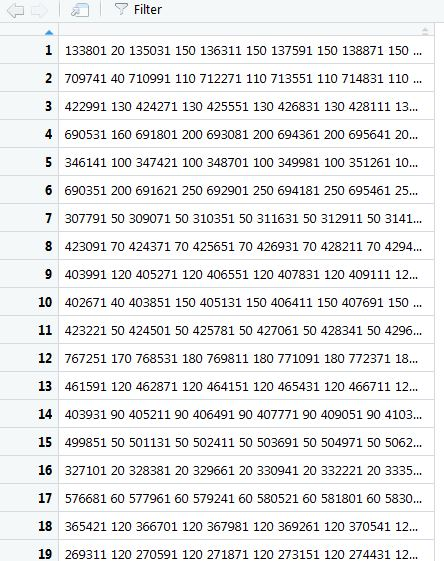GIF image for comparison of the original and predicted mask: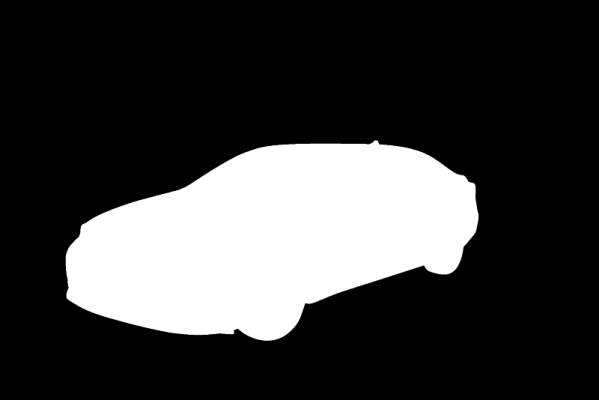The absence of small details is explained by the use of low-resolution images — only 128x128. When working in a higher resolution, the result will, of course, be better. With a shortage of memory, you can make predictions in batches of several thousand observations, and then save them in one file.

## Conclusion

In this article, it was shown that while sitting on R, one can keep up with fashion trends and successfully train deep neural networks. And even the Windows OS cannot stop it!

Topics:
keras ,deep learning ,ai ,tutorial ,r ,image recognition ,magick ,neural networks

Comment (0)

Save
{{ articles.views | formatCount}} Views

Opinions expressed by DZone contributors are their own.